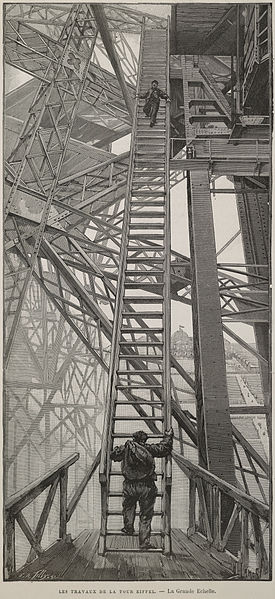# An interesting question and simulation 01Question: A man is standing on an infinite ladder. In one move, he can either go up (with probability 0.5), or stay where he is (with probability 0.5). The question is: What is the number of moves the man need to take to move 100 steps up from his initial position?

Let's transform this question to a math question:

For any $t>0$, $X_t$ is $i.i.d.$ Bernolli random variable with distribution

\begin{equation} X_{t} = \begin{cases} 1, & \text{ prob = 0.5}\\ 0, & \text{ prob = 0.5} \end{cases} \end{equation}

So we have $E(X) = \frac{1}{2}$ and $V(x) = \frac{1}{4}$

Let us denote $Y_n = \sum_{t=1}^{n}X_t$. Then $Y_n$ has binomial distribution $Bin \left(n, \frac{1}{2} \right)$.

Also, from Central Limit Theorem, we have $Y_n \sim \left(\frac{n}{2}, \frac{n}{4} \right)$.

So for the queston that the man will be 100 steps from his initial position means $Y_n \ge 100$.

Since $Y_n$ is random, I think the question should be changed to What is the number of moves the man need to take to move 100 steps up from his initial position with 95% confidence?

Then the question becomes a math question

\begin{aligned} &P(Y_n \ge 100) = 0.95 \\ & \Rightarrow P(Y_n \le 100) = 0.05 \\ & \Rightarrow P\left( \frac{Y_n - \frac{n}{2}}{\sqrt{\frac{n}{4}}} \le \frac{100 - \frac{n}{2}}{\sqrt{\frac{n}{4}}} \right) = 0.05 \\ & \Rightarrow P\left( Z \le \frac{100 - \frac{n}{2}}{\sqrt{\frac{n}{4}}} \right) = 0.05 \\ & \Rightarrow \frac{100 - \frac{n}{2}}{\sqrt{\frac{n}{4}}} = Z_{0.05} = -1.645 \\ & \Rightarrow n = 224.66 \end{aligned}

That is, we have 95% confidence that after 224 moves the man will be 100 steps up from his initial position.

We can simulate this in python with these steps:

1. simulate 10000000 randon variables with 0.5 prob equal to 1, and 0.5 prob equal to 0
2. calculate cumulative sum of these 10000000 simulated values
3. cut them by 100, 200, 300, 400, 500, ... and so on
4. count how many data points are there in each cut
5. this count is the number of steps for random walk reaching 100
6. calculate its percentile
import numpy as np
import pandas as pd

x = np.random.randint(0, 2, 10000000)
y = np.cumsum(x)
z = np.digitize(y, bins = (np.arange(10000000))*100)
w = pd.Series(z).groupby(z).size()
np.percentile(w, 95)

224.0


Most of the time we saw the question asked in this way: if that man moves 200, what is the expected steps he is away from the original position. This question is very easy and we know the answer is 100. It is the expection of $Y_{200}$, $E(Y_{200}) = 100$ .